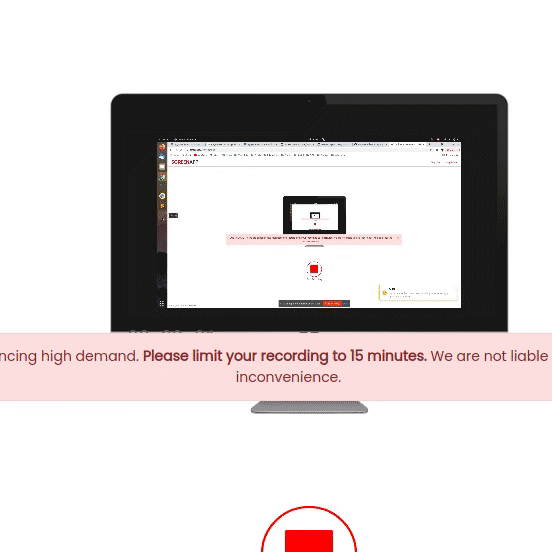# Pygame – Control Sprites

• Last Updated : 31 Jan, 2022

In this article, we will discuss how to control the sprite, like moving forward, backward, slow, or accelerate, and some of the properties that sprite should have. We will be adding event handlers to our program to respond to keystroke events, when the player uses the arrow keys on the keyboard we will call our pure methods to move the object on the screen.

## Functions Used

• moveRight(): This method takes argument pixels, which means how many pixels an object should be moved in the right direction. It is basically adding pixels to the current x coordinate of the object.
• moveLeft(): This method takes argument pixels, which means how many pixels an object should be moved in the left direction. It is basically subtracting pixels to the current x coordinate of the object.
• moveForward(): This method takes an argument speed, which means by how many factors the speed will increase. It is basically increasing speed with the factor of n in the y-direction of the object.
• moveBackward(): This method takes an argument speed, which means by how many factors the speed will decrease. It is basically decreasing speed with the factor of n in the y-direction of the object.

Let us first look at the implementation of a Sprite class, which helps us create an object on our PyGame surface, and along with added 4 methods that will help us move forward, backward, right, and left.

Example: Sprite class

## Python3

 `import` `pygame` `# GLOBAL VARIABLES``COLOR ``=` `(``255``, ``100``, ``98``)``SURFACE_COLOR ``=` `(``167``, ``255``, ``100``)``WIDTH ``=` `500``HEIGHT ``=` `500` `# Object class``class` `Sprite(pygame.sprite.Sprite):``    ``def` `__init__(``self``, color, height, width):``        ``super``().__init__()` `        ``self``.image ``=` `pygame.Surface([width, height])``        ``self``.image.fill(SURFACE_COLOR)``        ``self``.image.set_colorkey(COLOR)` `        ``pygame.draw.rect(``self``.image,``                         ``color,``                         ``pygame.Rect(``0``, ``0``, width, height))` `        ``self``.rect ``=` `self``.image.get_rect()` `    ``def` `moveRight(``self``, pixels):``        ``self``.rect.x ``+``=` `pixels` `    ``def` `moveLeft(``self``, pixels):``        ``self``.rect.x ``-``=` `pixels` `    ``def` `moveForward(``self``, speed):``        ``self``.rect.y ``+``=` `speed ``*` `speed``/``10` `    ``def` `moveBack(``self``, speed):``        ``self``.rect.y ``-``=` `speed ``*` `speed``/``10`

Now we will see how we have control of our main program loop to handle the sprites. The first part of the loop will respond to the events such as interactions when the user uses the mouse or the keyboard. Later, on above methods for event handling on our object will be taken care of. Each event handler will call the relevant method from the Sprite class.

In this piece of code, we have control of our object, i.e., our object is an object as per our given directions, if we press the right arrow key, it will move in that direction and the same with all the arrow keys. Here, we use pygame.KEYDOWN method to initialize the method to use the arrow keys for controlling the objects, later on, we have to control the respective method to trigger the specific key to perform the certain action.

For instance, if we have a right arrow key, we have to call pygame.K_RIGHT method to move towards right in the direction of the object, and similar for pygame.K_DOWN method which is used for the move up in the direction of the object.

Example: Controlling sprite

## Python3

 `import` `random``import` `pygame` `# Global Variables``COLOR ``=` `(``255``, ``100``, ``98``)``SURFACE_COLOR ``=` `(``167``, ``255``, ``100``)``WIDTH ``=` `500``HEIGHT ``=` `500` `# Object class``class` `Sprite(pygame.sprite.Sprite):``    ``def` `__init__(``self``, color, height, width):``        ``super``().__init__()` `        ``self``.image ``=` `pygame.Surface([width, height])``        ``self``.image.fill(SURFACE_COLOR)``        ``self``.image.set_colorkey(COLOR)` `        ``pygame.draw.rect(``self``.image,``                         ``color,``                         ``pygame.Rect(``0``, ``0``, width, height))` `        ``self``.rect ``=` `self``.image.get_rect()` `    ``def` `moveRight(``self``, pixels):``        ``self``.rect.x ``+``=` `pixels` `    ``def` `moveLeft(``self``, pixels):``        ``self``.rect.x ``-``=` `pixels` `    ``def` `moveForward(``self``, speed):``        ``self``.rect.y ``+``=` `speed ``*` `speed``/``10` `    ``def` `moveBack(``self``, speed):``        ``self``.rect.y ``-``=` `speed ``*` `speed``/``10`  `pygame.init()`  `RED ``=` `(``255``, ``0``, ``0``)`  `size ``=` `(WIDTH, HEIGHT)``screen ``=` `pygame.display.set_mode(size)``pygame.display.set_caption(``"Creating Sprite"``)`  `all_sprites_list ``=` `pygame.sprite.Group()` `playerCar ``=` `Sprite(RED, ``20``, ``30``)``playerCar.rect.x ``=` `200``playerCar.rect.y ``=` `300`  `all_sprites_list.add(playerCar)` `exit ``=` `True``clock ``=` `pygame.time.Clock()` `while` `exit:``    ``for` `event ``in` `pygame.event.get():``        ``if` `event.``type` `=``=` `pygame.QUIT:``            ``exit ``=` `False``        ``elif` `event.``type` `=``=` `pygame.KEYDOWN:``            ``if` `event.key ``=``=` `pygame.K_x:``                ``exit ``=` `False` `    ``keys ``=` `pygame.key.get_pressed()``    ``if` `keys[pygame.K_LEFT]:``        ``playerCar.moveLeft(``10``)``    ``if` `keys[pygame.K_RIGHT]:``        ``playerCar.moveRight(``10``)``    ``if` `keys[pygame.K_DOWN]:``        ``playerCar.moveForward(``10``)``    ``if` `keys[pygame.K_UP]:``        ``playerCar.moveBack(``10``)` `    ``all_sprites_list.update()``    ``screen.fill(SURFACE_COLOR)``    ``all_sprites_list.draw(screen)``    ``pygame.display.flip()``    ``clock.tick(``60``)` `pygame.quit()`

Output:My Personal Notes arrow_drop_up